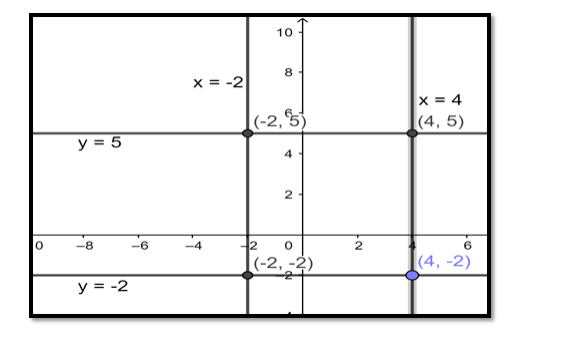# The sides of a rectangle are given by the equations`
Question:

The sides of a rectangle are given by the equations x = - 2, x = 4, y = - 2 and y = 5. Find the equation of the circle drawn on the diagonal of this rectangle as its diameter.

Solution:

The intersection points in clockwise fashion are:( - 2, 5), (4, 5), (4, - 2), ( - 2, - 2).

The equation of a circle passing through the coordinates of the end points of diameters is

$\left(x-x_{1}\right)\left(x-x_{2}\right)+\left(y-y_{1}\right)\left(y-y_{2}\right)=0$

Substituting, values: $\left(x_{1}, y_{1}\right)=(-2,5) \&\left(x_{2}, y_{2}\right)=(4,-2)$

We get:

$(x+2)(x-4)+(y-5)(y+2)=0$

$\Rightarrow x^{2}-4 x+2 x-8+y^{2}+2 y-5 y-10=0$

$\Rightarrow x^{2}+y^{2}-2 x-3 y-18=0$Ans: $x^{2}+y^{2}-2 x-3 y-18=0$